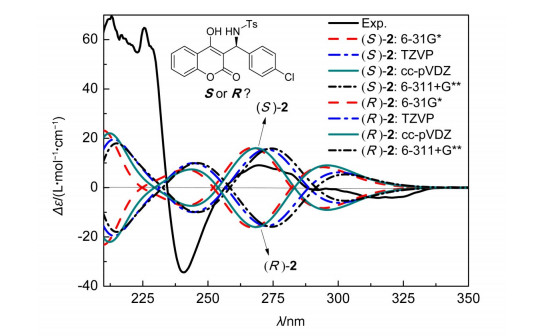## Determination of Absolute Configuration of Chiral Compounds Based on Chiroptical Spectroscopic Methods: From Instrument Characterization to Computational Chemistry

1,,2

 基金资助: 国家自然科学基金.  21103023

 Fund supported: 国家自然科学基金.  21103023Abstract

The determination of the absolute configuration (AC) of chiral compounds, especially the new chiral compounds, is one of the difficulties of the structure determination.The most widely used AC determination chiroptical spectroscopic methods include electronic and vibrational circular dichroism, optical rotatory dispersion, Raman optical activity etc.This mini review summarizes the main test principles, applications and corresponding theoretical calculation methods.With the developments of theoretical chemistry, the AC determination through instrumental characterization technique coupled with quantum chemical calculation, is envisaged to be the new trends of AC assignment.

Keywords： Absolute configuration ; Theoretical and computational chemistry ; Electronic circular dichroism ; Vibrational circular dichroism ; Optical rotatory dispersion

WANG Juan, YANG Xiao-Di. Determination of Absolute Configuration of Chiral Compounds Based on Chiroptical Spectroscopic Methods: From Instrument Characterization to Computational Chemistry. University Chemistry[J], 2016, 31(11): 37-44 doi:10.3866/PKU.DXHX201603022

### 图1### 1.1 电子圆二色谱

ECD法由于其干扰少，测试过程快捷简便，图谱包含信息量大，且发展总结了相应的图谱与构型对应的半经验规律，被广泛应用在手性化合物的测定上。随着量子化学理论的发展和计算机技术的进步，ECD谱可通过纯理论计算方法进行模拟，为通过ECD测量鉴定手性化合物的绝对构型提供了可行性

${{R}_{k}}=2.297\times {{10}^{-39}}\int \frac{\Delta {{\varepsilon }_{k}}(\lambda )\text{d}\lambda }{\lambda }\approx \frac{2.297\times {{10}^{-39}}}{{{\lambda }_{k}}}\text{ }\int \Delta {{\varepsilon }_{k}}(\lambda )\text{d}\lambda$

${{R}_{k}}=\operatorname{Im}\{\left\langle {{\mathit{\Psi }}_{0}}|\overset\frown{\mu }|{{\mathit{\Psi }}_{k}} \right\rangle \left\langle {{\mathit{\Psi }}_{0}}|\overset\frown{m}|{{\mathit{\Psi }}_{k}} \right\rangle \}$

$\Delta \varepsilon \left( E \right)=\frac{1}{2.297\times {{10}^{-39}}}\frac{1}{\sqrt{\text{ }\!\!\pi\!\!\text{ }}\sigma }\sum\limits_{k}{\Delta {{E}_{k}}{{R}_{k}}{{\text{e}}^{-{{[\left( E-\Delta {{E}_{k}} \right)/\sigma]}^{2}}}}}$

### 1.2 振动圆二色谱

VCD描述的是手性化合物在红外光谱区左旋圆偏振光和右旋圆偏振光的吸收系数之差随波长的变化关系。因此VCD的计算步骤与ECD类似，差别在于VCD在理论计算时考查的是化合物的振动吸收，不涉及激发态信息。对于第j正则模式对应的振动跃迁，对应的转动强度Rj定义为

${{R}_{j}}=\operatorname{Im}\{\left\langle {{\mathit{\Psi }}_{0, 0}}|\overset\frown{\mu }|{{\mathit{\Psi }}_{0, j}} \right\rangle \left\langle {{\mathit{\Psi }}_{0, j}}|\overset\frown{m}|{{\mathit{\Psi }}_{0, 0}} \right\rangle \}$

${{R}_{j}}=2.297\times {{10}^{-39}}\int \frac{\Delta {{\varepsilon }_{j}}(v)\text{d}v}{v}\approx \frac{2.297\times {{10}^{-39}}}{{{v}_{j}}}\text{ }\int \Delta {{\varepsilon }_{j}}(v)\text{d}v$

VCD谱通常用洛伦兹函数来展宽，根据Δε与振动频率v的关系，拟合出VCD谱线。

$\Delta \varepsilon \left( v \right)=\frac{1}{2.297\times {{10}^{-39}}}\frac{1}{\text{ }\!\!\pi\!\!\text{ }}\sum\limits_{j}{{{v}_{j}}{{R}_{j}}}\frac{\sigma }{{{\left( v-{{v}_{j}} \right)}^{2}}+{{\sigma }^{2}}}$

### 1.3 旋光光谱法

$\beta \left( \lambda \right)=\frac{1}{3}\left[{{\beta }_{xx}}\left( \lambda \right)+{{\beta }_{yy}}\left( \lambda \right)+{{\beta }_{zz}}\left( \lambda \right) \right]=\frac{4\text{ }\!\!\pi\!\!\text{ }c}{3h}\sum\limits_{k}{\frac{{{R}_{k}}}{\omega _{k}^{2}-{{\omega }^{2}}}}$

$\left[\chi \left( \lambda \right) \right]=[\alpha \left( \lambda \right)]\times \frac{M}{100}=0.915\times {{10}^{42}}\sum\limits_{k}{\frac{\lambda _{k}^{2}}{{{\lambda }^{2}}-\lambda _{k}^{2}}{{R}_{k}}}$

$\left[\chi \left( \lambda \right) \right]=\frac{0.915\times {{10}^{42}}}{2}\sum\limits_{k}{{{\lambda }_{k}}{{R}_{k}}}\left[\frac{\lambda-{{\lambda }_{k}}}{{{\left( \lambda-{{\lambda }_{k}} \right)}^{2}}+{{\sigma }^{2}}}-\frac{\lambda +{{\lambda }_{k}}}{{{\left( \lambda +{{\lambda }_{k}} \right)}^{2}}+{{\sigma }^{2}}} \right]$

#### 1.4.2 旋光拉曼光谱法

ROA的机理源于电偶极矩、磁偶极矩和电四偶极矩之间的耦合，其中的磁性来源于分子振动时产生的电荷流动所引起的磁场。ROA所测量的是比经过偶极矩过程的光吸收、光的散射更深的层次，涉及的是分子内跃迁的磁过程和四极矩过程；反映的是传统红外和常规拉曼技术无法提供的、更高层次的分子内部立体结构信息。目前理论层面的ROA计算主要是针对分子的电偶极-电偶极相互作用[22, 23]和电偶极-电四偶极相互作用。电偶极-磁偶极相互作用的计算机制仍有待完善

### 2.1 (4S, 5R, 6R)-oxysporone的绝对构型确定

(4S, 5R, 6R)-oxysporone (化合物1，如图2所示)是自尖刀镰孢菌中分离出的天然产物，目前并无X射线单晶衍射数据证明化合物1的绝对构型，其相对构型是通过NMR测试确定的。

### 图2Mazzeo等首先使用分子力学方法对化合物1的对映异构体分别进行构象搜索，获得6种优势构象。将这些构象在DFT/B3LYP/cc-pVTZ水平上做结构优化，确定各个构象的分布率。分别在EtOH和CHCl3溶液中，测试了化合物1的ORD谱(图3(a))，ORD谱较为平坦且呈正值。基于优化出的几何结构，在TDDFT/B3LYP/aug-cc-pVDZ水平上对化合物1进行ORD计算，并按照分构象分布率进行加和得到理论预测谱图。发现(4S, 5R, 6R)-1的ORD计算结果与实测结果更接近，(4R, 5S, 6S)-1的谱线与实验测得的[α]绝对值相近，但ORD谱线与实测谱线互为镜像。这表明(4S, 5R, 6R)-1更“符合”化合物1的绝对构型。

### 图3ECD谱(图3(b))是在MeCN (8.6 × 10-3 mol·L-1)溶液中测量的。测量的数据显示：化合物1在230 nm处有一低振幅、负Cotton效应(Cotton effect，CE)峰，在200 nm处对应着一个高强度的正CE峰。ECD是在TDDFT/CAM-B3LYP/aug-cc-pVDZ水平上计算的，计算了前30个激发态的信息，半峰宽设定为0.4 eV。将理论计算数据与实验测量数据作对比，发现(4S, 5R, 6R)-1的ECD谱线与实验值的符号和趋势均十分接近，进一步证明了化合物的绝对构型为(4S, 5R, 6R)-1

VCD计算是在B3LYP/6-31G (d)水平上，对各构象分别计算并按照其分布率加和获得的。VCD谱图(图4)中，各个峰的位置与在同一计算水平下预测的IR谱的峰位是一致的，并可通过频率计算将IR谱的各个吸收峰与振动模式作对照分析。(4R, 5S, 6S)-1和它的反型(4S, 5R, 6R)-1，它们的理论预测VCD谱图峰位一致，符号相反。VCD谱的分析结果与ORD和ECD是一致的。再一次确定了化合物1的绝对构型是(4S, 5R, 6R)-1

### 图4### 2.2 α-benzylamino-coumarins的绝对构型确定

α-benzylamino-coumarins (化合物2)可通过Mannich反应合成，产率高达99%，对映选择性达到83% ee。但其单晶难以培养，无法直接通过单晶衍射测试判断绝对构型。其衍生物培养出的单晶结构经X射线衍射测试后，发现晶胞中RS构型交替排列，无法通过衍生物的相对构型反推化合物2的绝对构型。故采用ECD测试并结合理论预测的方法来确定手性化合物的绝对构型。针对RS型的化合物2，首先使用Confab程序进行构象搜索，对能量间隔在0-83.7 kJ·mol-1的几何结构做计算水平为B97D/6-31G*的结构优化并按照能量高低排序。以12.6 kJ·mol-1为能量截点对剩余的分子构象做DFT/B3LYP/TZVP结构优化，并考虑PCM溶剂效应，获得各个构象的Boltzmann分布和分子几何。激发态信息采用B3LYP泛函，并选择不同的基组如6-31G*、TZVP、cc-pVDZ、6-311+G**进行比较计算。理论预测表明S型的ECD谱其峰位和符号与实验测试谱图完全吻合(图5)，R型的ECD谱图与S型峰位一致，符号相反。因此化合物2的绝对构型为S型。

### 图5## 参考文献 原文顺序 文献年度倒序 文中引用次数倒序 被引期刊影响因子

Cheong P.H.Y. ; Legault C.Y. ; Um J.M. ; Celebi-Olcum N. ; Houk K.N. Chem.Rev. 2011, 111 (8), 5042.

Bijvoet J.M. ; Peerdeman A.F. ; van Bommel A.J. Nature 1951, 168 (4268), 271.

Lightner D.A. ; Gurst J.E. Wiley-VCH: New York, Organic Conformational Analysis and Stereochemistry from Circular Dichroism Spectroscopy 2000.

Berova N. ; Di Bari L. ; Pescitelli G. Chem.Soc.Rev. 2007, 36 (6), 914.

Freedman T.B. ; Cao X.L. ; Dukor R.K. ; Nafie L.A. Chirality 2003, 15 (9), 743.

Diedrich C. ; Grimme S.J. Phys.Chem.A 2003, 107 (14), 2524.

Bak K.L. ; Hansen A.E. ; Ruud K. ; Helgaker T. ; Olsen J. ; Jorgensen P. Theor.Chim.Acta 1995, 90 (5-6), 441.

Grimme S. Chem.Phys.Lett. 1996, 259 (1-2), 128.

Stephens P.J. ; Devlin F.J. ; Pan J.J. Chirality 2008, 20 (5), 634.

Polavarapu P.L. Chem.Rec. 2007, 7 (2), 125.

Frisch, M.J.T.; Schlegel, G.W.; Scuseria, H.B.; Robb, G.E.; Cheeseman, M.A.; Montgomery, J.R.; et al.Gaussian 09, Revision B.01;Gaussion Inc.:Wallingtord, CT, 2009.

Polavarapu P.L. Chirality 2008, 20 (5), 664.

Giorgio E. ; Viglione R.G. ; Zanasi R. ; Rosini C.J. Am.Chem.Soc. 2004, 126 (40), 12968.

Polavarapu P.L. Chirality 2006, 18 (5), 348.

Harada N. ; Nakanishi K. Acc.Chem.Res. 1972, 5 (8), 257.

Gawronski J. ; Skowronek P. Curr.Org.Chem. 2004, 8 (1), 65.

Tanaka K. ; Pescitelli G. ; Nakanishi K. ; Berova N. Monatsh.Chem. 2005, 136 (3), 367.

Barron L.D. ; Buckingham A.D. Mol.Phys. 1971, 20 (6), 1111.

Polavarapu P.L.J. Phys.Chem. 1990, 94 (21), 8106.

Polavarapu P.L. ; Hecht L. ; Barron L.D.J. Phys.Chem. 1993, 97 (9), 1793.

Frisch M.J. ; Yamaguchi Y. ; Gaw J.F. ; Schaeffer Ⅱ H.F. ; Binkley J.S.J. Chem.Phys. 1986, 84 (1), 531.

Quinet O. ; Champagne B.J. Chem.Phys. 2001, 115 (14), 6293.

Quinet O. ; Liegeois V. ; Champagne B.J. Chem.Theory Comput. 2005, 1 (3), 444.

Mazzeo G. ; Santoro E. ; Andol A. ; Cimmino A. ; Troselj P. ; Petrovic A.G. ; Superchi S. ; Evidente A. ; Berova N.J. Nat.Prod. 2013, 76 (4), 588.

Lin H. ; Tan Y. ; Wu J.M. ; Yang X.D. ; Chen J.H. ; Sun X.W. Tetrahedron Lett. 2015, 56 (7), 913.

/

 〈〉